Our prices are denominated in USD.

We ship internationally!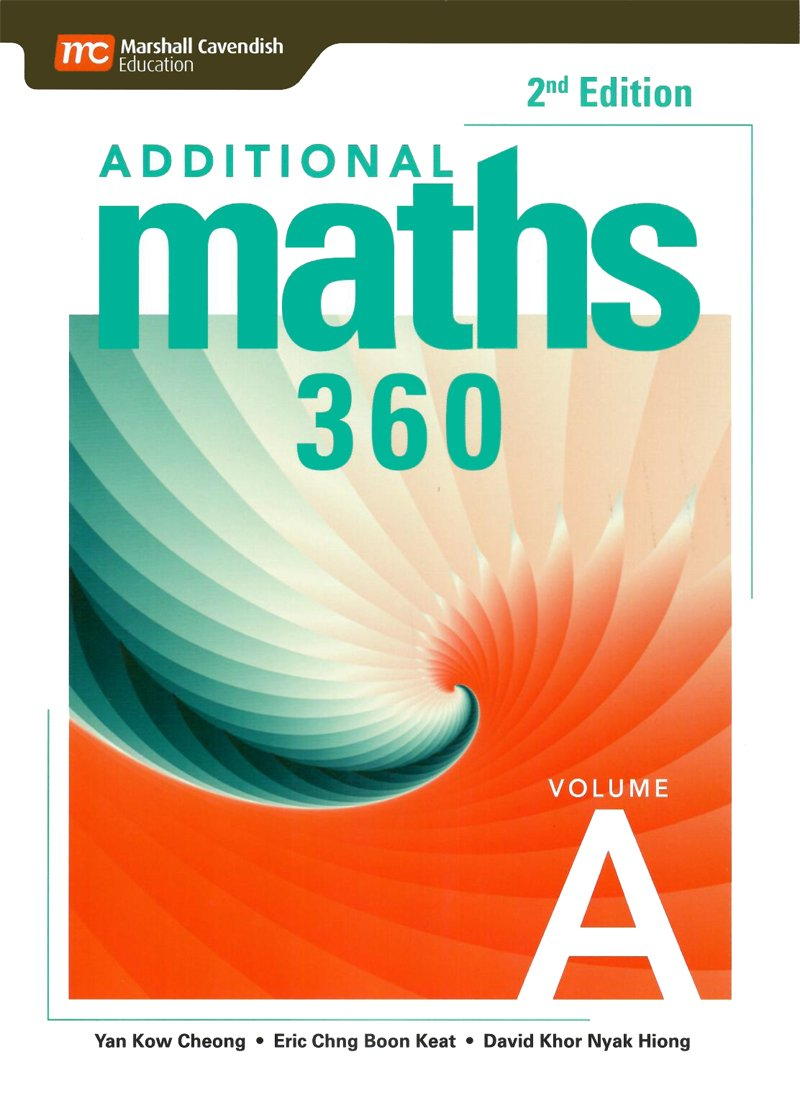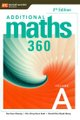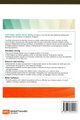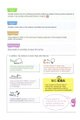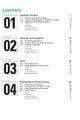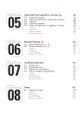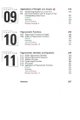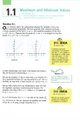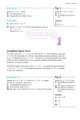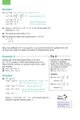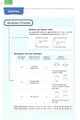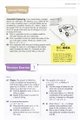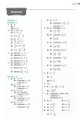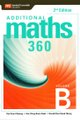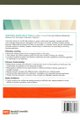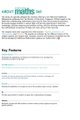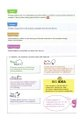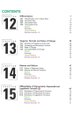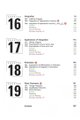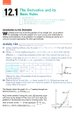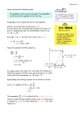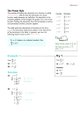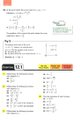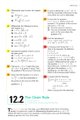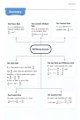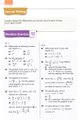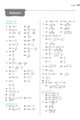US\$19.35

This two-book series is suitable for 2 or 3 year programme.

This series stimulates students' interest in learning mathematical concepts and helps them develop thinking, reasoning, metacognitive and problem-solving skills required for higher studies in mathematics and other disciplines. Various mathematical concepts and ideas are used cohesively to help learners connect maths to other subjects through the application of maths, develop deep understanding of maths and appreciate maths in its abstract form.

Features:

• New Trend Questions
Real-world contextual, application and modelling questions and Exam-based worked examples

• Levelled Questions
Classified according to difficulty levels so students can assess their competency level

• Extend Section
Provides students with more challenging real-world problems, involving concepts that have not yet been formally covered. The sections also provide opportunities for students to do research and write computer programs.

Topics in Volume A:

2. Equations and Inequalities
3. Surds
4. Polynomials and Partial Fractions
5. Exponential and Logarithmic Functions
6. Binomial Theorem
7. Coordinate Geometry
8. Circles
9. Applications of Straight Line Graphs
10. Trigonometric Functions
11. Trigonometric Identities and  Equations

Topics in Volume B:

1. Differentiation
2. Tangents, Normals and Rates of Change
3. Maxima and Minima
4. Differentiation of Trigonometric, Exponential and Logarithmic Functions
5. Integration
6. Applications of Integration
7. Kinematics
8. Plane Geometry

Complete series contain:

1. Textbook
2. Extra Practice
3. Guided Books